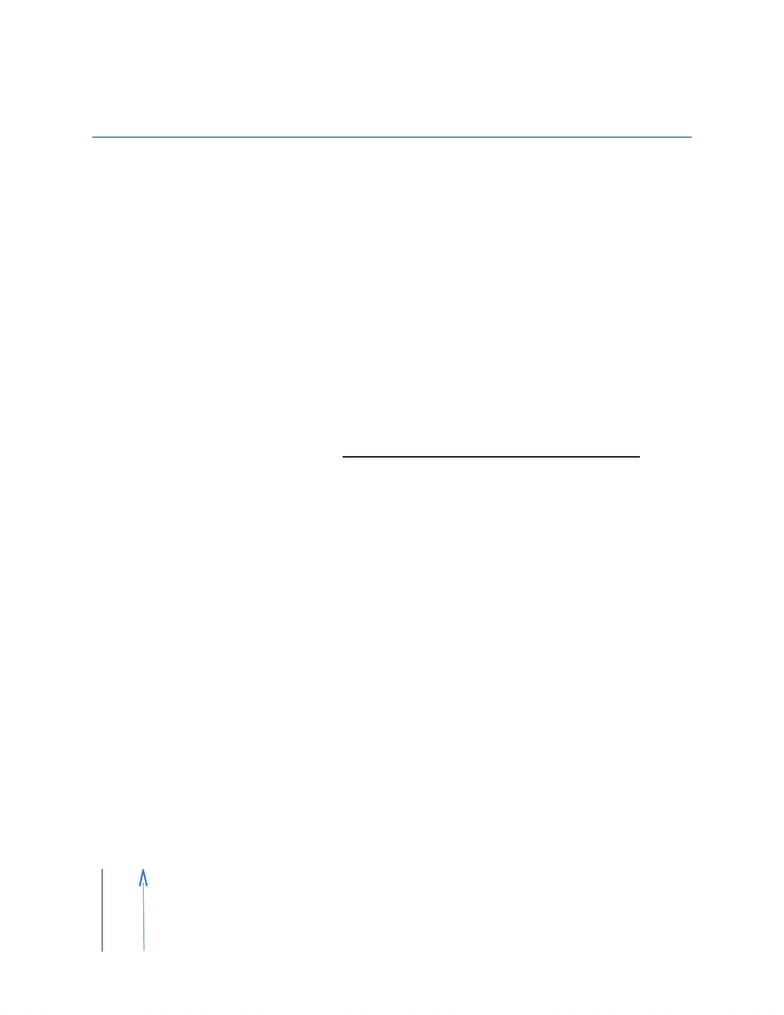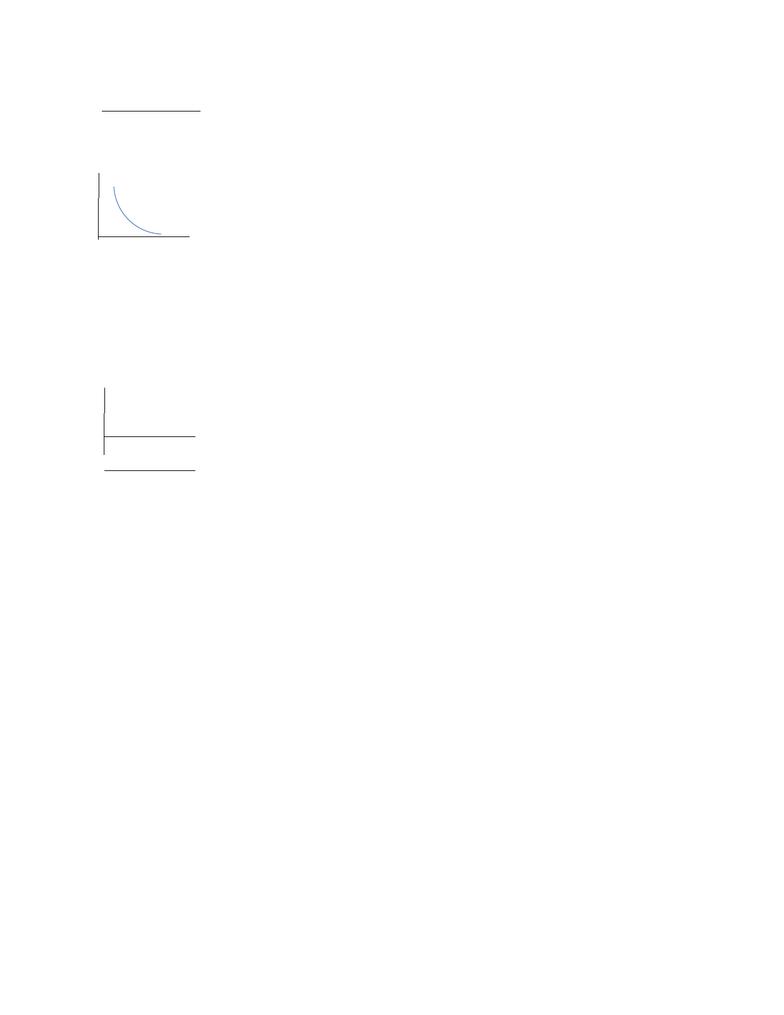# Economics 1021A/B Chapter Notes - Chapter 4: Negative Number, Demand Curve, Inferior Good

26 views6 pagesEconomics Chapter 4 Notes
October 2, 2010
Elasticity
When supply increases, the equilibrium price falls and the equilibrium
quantity increases
Does the price fall by a large amount and does the quantity increase by a
little? Does the price barely fall and the quantity increase by a large amount?
Depends on the responsiveness of the quantity demanded compared
to a change in price
Price elasticity of Demand: a units free measure of the responsiveness of the
quantity demanded of a good to a change in its price when all other influences on
Price elasticity of demand = percentage change in quantity demanded
Percentage change in price
To use this formula, we need to know the quantities demanded at different
prices when all other influences on buying plans remain the same
We express the changes in price and quantity demanded as percentages of
the average price and the average quantity
By using these we calculate the elasticity at the point on the demand
curve midway between the original point an a new point
E.g
original price is 20.50 and new price is 19.50, therefore, average price
is 20.00. 1.00 price decrease so.. (\$1/\$20) X 100 = 5%
original quantity demanded is 9 pizzas and the new is 11 pizzas,
therefore, average quantity demanded is 10. 2 pizza increase so…
(2/10) X 100 = 20%
price elasticity of demand = 20%/5% = 4.
When the price of a good rises, the quantity demanded decreases. Because
of a positive change in price brings a negative change in the quantity
demanded, the price elasticity of demand is a negative number. This is the
magnitude or absolute value.
Perfectly inelastic demand: demand with a price elasticity of zero; the quantity
demanded remains constant when the price changes
Unlock document

This preview shows pages 1-2 of the document.
Unlock all 6 pages and 3 million more documents.Unit Elastic Demand: demand with a price elasticity of 1; the percentage change
in the quantity demanded equals the percentage change in price
Inelastic Demand: a demand with a price elasticity between 0 and 1; the
percentage change in quantity demanded is less than the percentage change in
price
Perfectly elastic Demand: demand with an infinite price elasticity; the quantity
demanded changes by an infinitely large percentage in response to a tiny price
change
E.g. a soft drink from two campus machines located side by side. Same soft
drinks for the same price, people buy from both. If one has a higher price,
even by a small amount, no one will buy from the other one therefore
showing perfectly elastic demand.
Total revenue: from a sale of a good equals the price of the good multiplied by the
quantity sold
When price changes, total revenue also changes but a rise in price does not
always increase totally revenue. It depends on the elasticity of demand in the
following way:
In demand is elastic, a 1 percent price cut increase the quantity sold
by more than 1 percent and total revenue increases
If demand is inelastic, a 1 percent price cut increases the quantity sold
by less than 1 percent and total revenue decreases
If demand is unit elastic, a 1 percent price cut increases the quantity
sold by 1 percent and total revenue does not change
Total Revenue Test: a method of estimating the price elasticity of demand by
observing the change in total revenue that results from a change in price, when all
other influences on the quantity sold remain the same
If a price cut increase total revenue, demand is elastic
Unlock document

This preview shows pages 1-2 of the document.
Unlock all 6 pages and 3 million more documents.